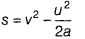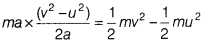# Prove that the increase in kinetic energy of the body is equal to the work done by the force on the body

The velocity of a body moving in a straight line is increased by applying a constant force F, for some distance in the direction of the motion. Prove that the increase in kinetic energy of the body is equal to the work done by the force on the body.

Consider an object of mass = m, uniform velocity = u and distance = s
Work done, (W) = F.s = ma.s …(1)
The work done on the object will cause a damage in its velocity. Let its velocity change from u to v.
v2 – u2 = 2asSubstituting the value of s in Eq. (1), we get
W = ma.s =Thus, work done is equal to the increase in kinetic energy.